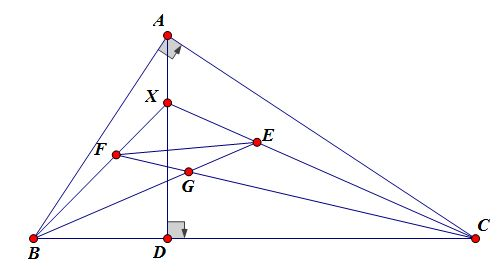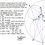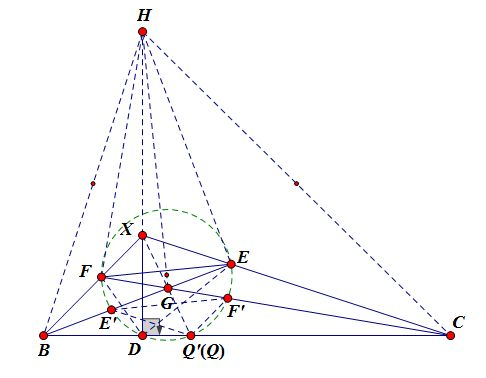# [Solutions Posted]Geometry Proof Problem of the day #2 (diagram included)

It's been a day or two? I did not keep track. Found this one in an online conversation I had with a geometer friend of mine; it might be or based on an existing olympiad problem though. I never keep track anyway.$\triangle ABC$ has a right angle at $A$, $D\in BC$ and $AD\perp BC$. $X$ is an arbitrary point of $AD$. $E,F\in CX,BX$ respectively such that $AB=BE,AC=CF$.

Suppose $BE\cap CF=G$. Prove that $FG=GE$.

Extra Credit: Let $XG\cap BC=Q$. Prove that $E,F,D,Q$ are concyclic.Note by Xuming Liang
4 years, 5 months ago

This discussion board is a place to discuss our Daily Challenges and the math and science related to those challenges. Explanations are more than just a solution — they should explain the steps and thinking strategies that you used to obtain the solution. Comments should further the discussion of math and science.

When posting on Brilliant:

• Use the emojis to react to an explanation, whether you're congratulating a job well done , or just really confused .
• Ask specific questions about the challenge or the steps in somebody's explanation. Well-posed questions can add a lot to the discussion, but posting "I don't understand!" doesn't help anyone.
• Try to contribute something new to the discussion, whether it is an extension, generalization or other idea related to the challenge.

MarkdownAppears as
*italics* or _italics_ italics
**bold** or __bold__ bold
- bulleted- list
• bulleted
• list
1. numbered2. list
1. numbered
2. list
Note: you must add a full line of space before and after lists for them to show up correctly
paragraph 1paragraph 2

paragraph 1

paragraph 2

[example link](https://brilliant.org)example link
> This is a quote
This is a quote
    # I indented these lines
# 4 spaces, and now they show
# up as a code block.

print "hello world"
# I indented these lines
# 4 spaces, and now they show
# up as a code block.

print "hello world"
MathAppears as
Remember to wrap math in $$ ... $$ or $ ... $ to ensure proper formatting.
2 \times 3 $2 \times 3$
2^{34} $2^{34}$
a_{i-1} $a_{i-1}$
\frac{2}{3} $\frac{2}{3}$
\sqrt{2} $\sqrt{2}$
\sum_{i=1}^3 $\sum_{i=1}^3$
\sin \theta $\sin \theta$
\boxed{123} $\boxed{123}$

Sort by:

The first part of this problem turns out is from IMO 2012.

- 4 years, 5 months ago

Solution 1:What is special about $E,F$ in relation to $D$? Well, we do know from right triangle $ABC$ that $BE^2=AB^2=BD\cdot DC, CF^2=AC^2=CD\cdot CB$, implying $BDE\sim BEC, CDF\sim CFB$.

Construct the orthocenter of $XBC$ denoted by $H$. Then $\angle BED=\angle XCB=\angle BHD\implies B,H,D,E$ are concyclic. Since $BH\cap CX, \angle HEB=\angle HDB=90^{\circ}$, we can easily deduce that $HE^2=HX\cdot HD$(try extending $CX$ to meet $BH$). Likewise we can show $HF^2=HX\cdot HD$, so $HE=HF$. Since $\angle HEG=\angle HFG=90^{\circ}$, therefore $GE=GF$.

Extra Credit: Let $\odot DEF\cap BC=D,Q'$, we will show that $Q'\in XG$ which implies $Q\equiv Q'$.

Suppose $\odot DEF\cap BE,BF=E',F'$. Then $\angle EE'Q'=\angle EDQ'=\angle XEB\implies XE||E'Q'$. Analogously $Q'F'||FX$. Furthurmore, $FG=GE$ implies that $FEF'E'$ is an isosceles trapezoid. Hence $EF||E'F'$, establishing that $XEF, Q'E'F'$ are homothetic and thus $XQ'$ passes through the center of homothety which is $G$. We are done.

- 4 years, 5 months ago

Hey Xuming, please upload the solution. I am very eager to know about the proof. I tried alot but cant succeed. Atleast any HINT. :D

- 4 years, 5 months ago

Wish granted! Next time you could tag my name to get my attention faster.

- 4 years, 5 months ago

Thanks @Xuming Liang Nice solution.

- 4 years, 5 months ago

Nice one by revealing the hidden orthic configuration! :) :) :)

- 4 years, 5 months ago